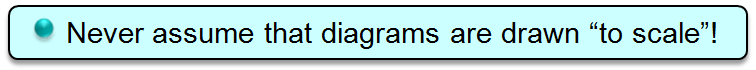Basic Geometric Symbols & Labeling MathBitsNotebook.com Terms of Use   Contact Person: Donna RobertsMathematics is a form of a language. And as such, it has its own manner of communicating information. As you progress in your mathematical career, you will discover that mathematics relies more and more on its own set of symbols when discussing information. Let's take a look at some of the geometry symbols we will be using. (For information on the correct notation, using these symbols, see Notation.)
 Geometric Symbol Interpretation Example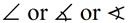Angle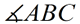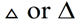Triangle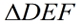capital letter Point point A ↔ Line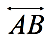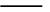Line Segment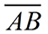→ or ← Ray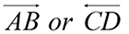|| Parallel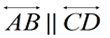⊥ Perpendicular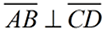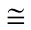Congruent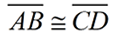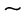Similar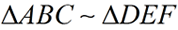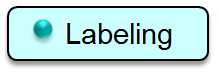Now, let's take a look at how figures are labeled in geometry, and the meanings of these labels.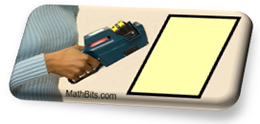Angles: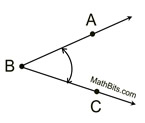or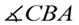Angles are labeled by specifying 3 points, with the center point being the vertex of the angle. This angle is NOT ∠BAC.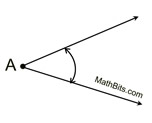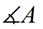Angles may be labeled with a single letter at the vertex, as long as it is perfectly clear that there is only one angle at this vertex.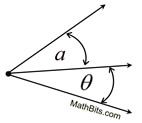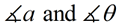Angles may be represented by a single lower case letter or by a Greek letter, as long as it is clear which angle is being referenced.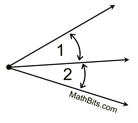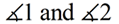Angles may also be represented by numbers, as long as it is clear to which angle the number applies.

Lines: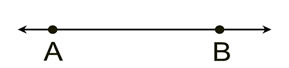Lines are traditionally labeled by expressing two points through which the line passes.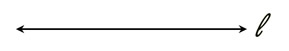line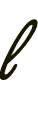Lines may also be labeled with a single scripted letter, and referred to by that name.

Closed Figures: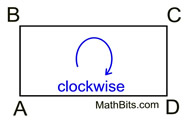When drawing rectangle ABCD: the letters must follow, in order, around the outside of the figure. You may start at any vertex point.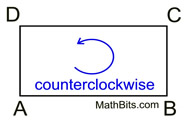When drawing rectangle ABCD: you may label in either a clockwise or counterclockwise direction around the outside of the figure.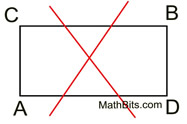When drawing rectangle ABCD: you may NOT label "across" the figure as shown here. This is not rectangle ABCD. (It is rectangle ACBD.)

Triangles: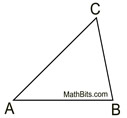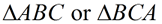or any other three letter combination of A, B and C will apply to this triangle.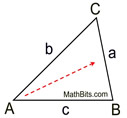When using letters to refer to the sides of a triangle, it is customary to label the sides as small case letters. Across from the vertex labeled capital A will be the side labeled small case a, and so on.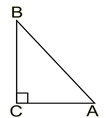A right triangle is designated with a "box" drawn in the location of the right angle.

Parallels and Perpendiculars: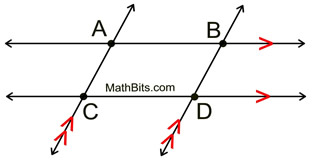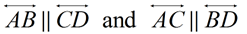Parallel lines are marked with "feathers". Notice how they appear as sets: the lines with one feather are parallels, and the lines with two feathers are parallel.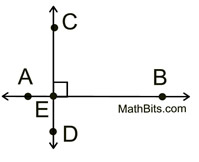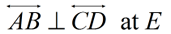A "box" at the point of intersection indicates the lines are perpendicular. This "box" represents the presence of a right angle (just as it did in the right triangle).

Congruent Sides and Angles: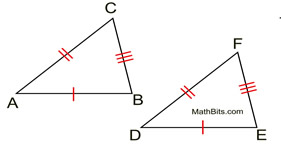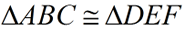"Hash marks" are used to represent segments of equal length on diagrams. Notice that corresponding sides are distinguished by their number of hash marks. It is customary, with congruent triangles, to "letter" the triangles' names in a corresponding order: vertex A will correspond to vertex D, vertex B to vertex E, and vertex C to vertex F, so triangle ABC is congruent to triangle DEF.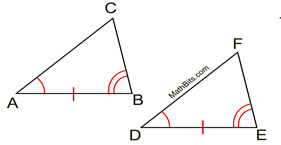While "hash marks" are used to represent segments of equal length on diagrams, "arcs" are used to represent angles of equal measure. In the same fashion as we saw with the hash marks, corresponding angles are distinguished by their number of arcs.